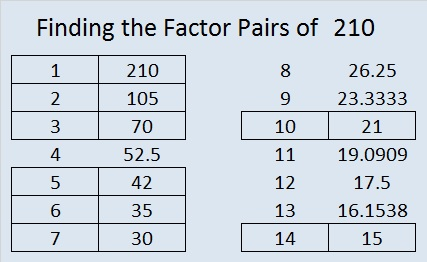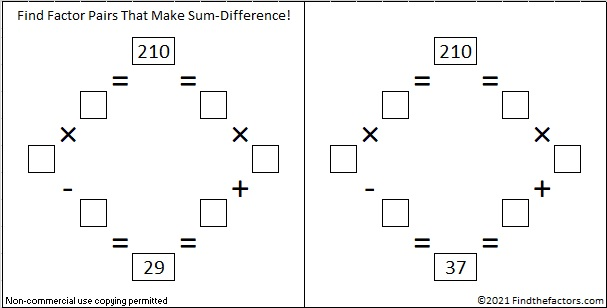# 210 and Level 4

### Today’s Puzzle:

Excel file of puzzles and previous week’s factor solutions: 10 Factors 2014-08-18

### Factors of 210:

• 210 is a composite number and a triangular number.
• Prime factorization: 210 = 2 x 3 x 5 x 7, so 210 is the product of the first four prime numbers!
• The exponents in the prime factorization are 1, 1, 1, and 1. Adding one to each and multiplying we get (1 + 1)(1 + 1)(1 + 1)(1 + 1) = 2 x 2 x 2 x 2 = 16. Therefore 210 has 16 factors.
• Factors of 210: 1, 2, 3, 5, 6, 7, 10, 14, 15, 21, 30, 35, 42, 70, 105, 210
• Factor pairs: 210 = 1 x 210, 2 x 105, 3 x 70, 5 x 42, 6 x 35, 7 x 30, 10 x 21, or 14 x 15
• Since 210 has no square factors, √210 cannot be simplified. √210 ≈ 14.491### Sum-Difference Puzzles:

210 has eight factor pairs. One of those factor pairs adds up to 29, and another one subtracts to 29. Another factor pair adds up to 37, and a different one subtracts to 37. Thus, 210 produces two different Sum-Difference Puzzles!210 is the smallest number that makes sum-difference two different ways!

### More about the Number 210:

210 is the hypotenuse of a Pythagorean triple:
126-168-210, which is (3-4-5) times 42.

Because 20 + 1 = 21 and (20/2) x 21 = 210, we know that 1 + 2 + 3 + 4 + 5 + 6 + 7 + 8 + 9 + 10 + 11 + 12 + 13 + 14 + 15 + 16 + 17 + 18 + 19 + 20 = 210, thus it is the 20th triangular number.

### A Logical Way to Solve Today’s Puzzle: